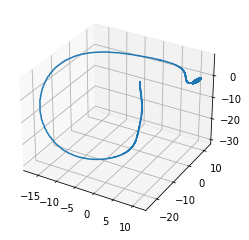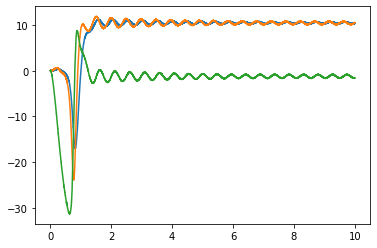# The Lorenz chaotic attractor¶

This example shows the construction of a classic chaotic dynamical system: the Lorenz “butterfly” attractor. The equations are:

$\begin{split}\dot{x}_0 = \sigma(x_1 - x_0) \\\ \dot{x}_1 = x_0 (\rho - x_2) - x_1 \\\ \dot{x}_2 = x_0 x_1 - \beta x_2\end{split}$

Since $$x_2$$ is centered around approximately $$\rho$$, and since NEF ensembles are usually optimized to represent values within a certain radius of the origin, we substitute $$x_2' = x_2 - \rho$$, giving these equations:

$\begin{split}\dot{x}_0 = \sigma(x_1 - x_0) \\\ \dot{x}_1 = - x_0 x_2' - x_1 \\\ \dot{x}_2' = x_0 x_1 - \beta (x_2' + \rho) - \rho\end{split}$

For more information, see http://compneuro.uwaterloo.ca/publications/eliasmith2005b.html “Chris Eliasmith. A unified approach to building and controlling spiking attractor networks. Neural computation, 7(6):1276-1314, 2005.”

:

%matplotlib inline
import matplotlib.pyplot as plt
from mpl_toolkits.mplot3d import Axes3D

import nengo

:

tau = 0.1
sigma = 10
beta = 8.0 / 3
rho = 28

def feedback(x):
dx0 = -sigma * x + sigma * x
dx1 = -x * x - x
dx2 = x * x - beta * (x + rho) - rho

return [
dx0 * tau + x,
dx1 * tau + x,
dx2 * tau + x,
]

model = nengo.Network(label="Lorenz attractor")
with model:
nengo.Connection(state, state, function=feedback, synapse=tau)
state_probe = nengo.Probe(state, synapse=tau)

with nengo.Simulator(model) as sim:
sim.run(10)

:

ax = plt.figure().add_subplot(111, projection=Axes3D.name)
ax.plot(*sim.data[state_probe].T)

plt.figure()
plt.plot(sim.trange(), sim.data[state_probe])

:

[<matplotlib.lines.Line2D at 0x7fbdf942b518>,
<matplotlib.lines.Line2D at 0x7fbdf942b4a8>,
<matplotlib.lines.Line2D at 0x7fbdf942b5f8>]